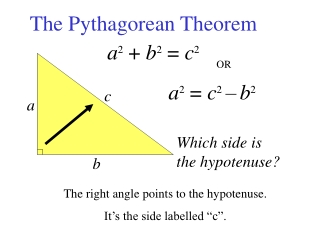DownloadDownload PresentationThe Pythagorean Theorem

# The Pythagorean Theorem

Download Presentation## The Pythagorean Theorem

- - - - - - - - - - - - - - - - - - - - - - - - - - - E N D - - - - - - - - - - - - - - - - - - - - - - - - - - -
##### Presentation Transcript

1. c a b The Pythagorean Theorem a2 + b2 = c2 OR a2 = c2–b2 Which side is the hypotenuse? The right angle points to the hypotenuse. It’s the side labelled “c”.

2. c 6 8 When calculating the hypotenuse, we add the area of the squares of the other two sides. Calculate side c. c2 = a2 + b2 c2 = 82+ 62 c2 = 64+ 36 c2 = 100 c = 10

3. x 7 12 Calculate the length of side x. b2 = c2– a2 x2 = 122– 72 a b x2 = 144– 49 x2= 95 c When calculating a side, we determine the difference of the area of the square of the hypotenuse and the area of the square of the known side. x= 9.7

4. 12 cm 15 cm The length and width of a rectangle are 12 cm and 15 cm. Calculate the length of the diagonal. c2 = a2+ b2 d 2 = 152+ 122 d d 2 = 225+ 144 d 2 = 369 d = 19.2 cm

5. 13 cm h 5 cm Tanya is making a party hat using a cone made out of paper. Determine the height of the cone. b2 = c2– a2 h2 = 132– 52 h2 = 169– 25 h2 = 144 h = 12 cm